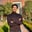Related Tags

python
communitycreator

# First class functions in PythonSaad Akbar

### Overview

A programming language, such as Python, enables the use of first-class functions if they fulfill the properties of first-class objects. First-class objects are those objects that we can deal with identically. These objects can be passed as parameters, used in control structures, or saved in data structures. First-class functions have the following properties:

### Example

In the example below, a function is treated as an object.

def learn(text):
return text

print (learn('Educative'))
#function treated as object

print (read('Educative'))

### Explanation

The read object is assigned the learn function as an object. The read object then inherits the functionality of the function as shown above.

### Example

In this example, a function is passed as an argument to another function.

def casual(text):
return text.lower()

def serious(text):
return text.upper()

def speak(func):
# function saved in a variable
speaks = func("""Learn from Educative""")
print (speaks)

speak(serious)
speak(casual)

### Explanation

The speak function is created where another function is passed in its parameter. For instance, passing serious will print the text in capital letters and casual will print it in small letters.

### Example

In this example, we return a function from another function.

def parent_multiply(a):
def child_multiply(b):
return a*b
#Parent returns child function
return child_multiply

multiply = parent_multiply(15)

print (multiply(2))

### Explanation

The parent_multiply function returns the child_multiply function. In this case, the child_multiply function calculates the product and parent_multiply function returns child_multiply function.

RELATED TAGS

python
communitycreator

CONTRIBUTORSaad Akbar
RELATED COURSES

View all Courses

Keep Exploring

Learn in-demand tech skills in half the time